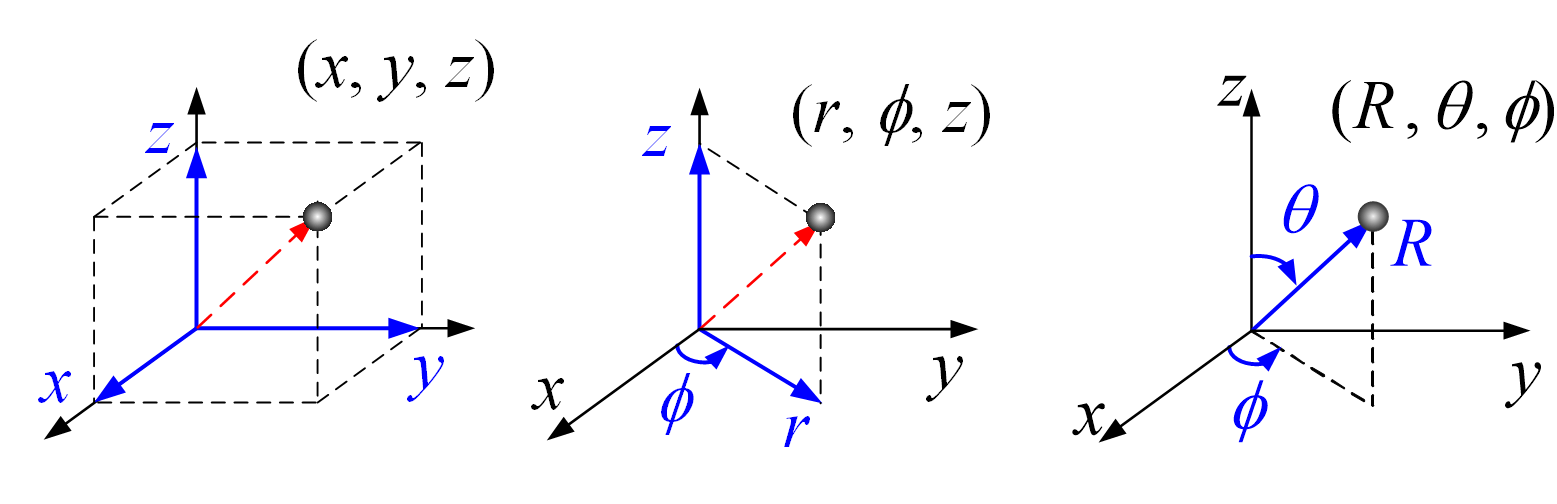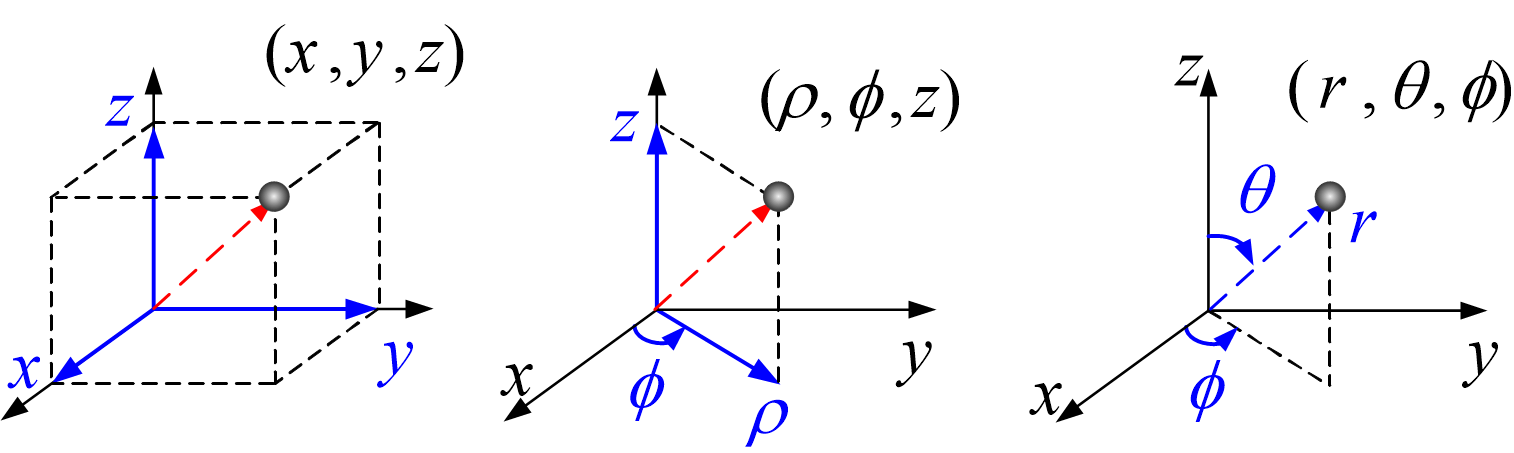Module 1.6 Relation between Unit Vectors of Various Othogonal Coordinate Systems

 Animation

 Cartesian Coordinates:    x = (m), y = (m), z = (m). Cylindrical Coordinates: r = (m), φ = o,     z = (m). Spherical Coordinates:    R = (m), θ = o,    φ = o.

### Introduction:

Axis X：Red arrow
Axis Y：Green arrow
Axis Z：Blue arrow
1. Press the left mouse button and move the mouse cursor to change the viewpoint, or you can click the standard view button in the upper right corner.
2. Press the right mouse button and move the graph with your mouse.

### Parameters:

Naming convention I:Cartesian coordinates     Cylindrical coordinates      Spherical coordinates
Naming convention II:Cartesian coordinates     Cylindrical coordinates      Spherical coordinates